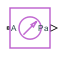# Pressure & Temperature Sensor (G)

Measure pressure and temperature differences

• Library:
• Simscape / Foundation Library / Gas / Sensors

•## Description

The Pressure & Temperature Sensor (G) block represents an ideal sensor that measures pressure and temperature in a gas network. There is no mass or energy flow through the sensor.

The physical signal ports P and T report the pressure difference and the temperature difference, respectively, across the sensor. The measurements are positive when the values at port A are greater than the values at port B.

To measure the absolute pressure and absolute temperature at port A, connect port B to an Absolute Reference (G) block.

Temperature measurements are different from pressure measurements, because the energy flow rate depends on the flow direction. The temperature measurement represents the temperature upstream of the measured node. For example, if port A of the Pressure & Temperature Sensor (G) is connected to a node between pipe 1 and pipe 2 and the gas flows from pipe 1 to pipe 2, then the temperature measured at port A is the temperature of the gas volume in pipe 1. If the gas then switches direction and flows from pipe 2 to pipe 1, then the temperature measured at port A is the temperature of the gas volume in pipe 2. If the temperatures of the two gas volumes are different, then this will manifest as a change in the measured temperature when the flow direction switches. If there are two or more upstream flow paths merging at the node, then the temperature measurement at the node represents the weighted average based on the ideal mixing of the merging flow.

## Ports

### Output

expand all

Physical signal output port for pressure difference measurement.

Physical signal output port for temperature difference measurement.

### Conserving

expand all

Gas conserving port. The measurements are positive when the values at port A are greater than the values at port B.

Gas conserving port. The measurements are positive when the values at port A are greater than the values at port B.

## Version History

Introduced in R2016b